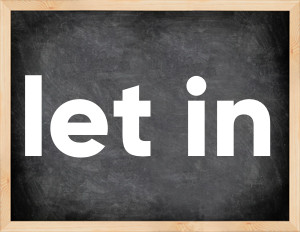# Phrasal verb let inThe English verb 'let in' is pronounced as [let ɪn].
Related to: phrasal verb.
3 forms of verb let in: Infinitive (let in), Past Simple - (let in), Past Participle - (let in).

## Here are the past tense forms of the verb let in

👉 Forms of verb let in in future and past simple and past participle.
❓ What is the past tense of let in.

## Let in: Past, Present, and Participle Forms

Base Form Past Simple Past Participle
let in [let ɪn]

let in [let ɪn]

let in [let ɪn]

## What are the 2nd and 3rd forms of the verb let in?

🎓 What are the past simple, future simple, present perfect, past perfect, and future perfect forms of the base form (infinitive) 'let in'?

### Learn the three forms of the English verb 'let in'

• the first form (V1) is 'let in' used in present simple and future simple tenses.
• the second form (V2) is 'let in' used in past simple tense.
• the third form (V3) is 'let in' used in present perfect and past perfect tenses.

## What are the past tense and past participle of let in?

The past tense and past participle of let in are: let in in past simple is let in, and past participle is let in.

### What is the past tense of let in?

The past tense of the verb "let in" is "let in", and the past participle is "let in".

### Verb Tenses

Past simple — let in in past simple let in (V2).
Future simple — let in in future simple is let in (will + V1).
Present Perfect — let in in present perfect tense is let in (have/has + V3).
Past Perfect — let in in past perfect tense is let in (had + V3).

### let in regular or irregular verb?

👉 Is 'let in' a regular or irregular verb? The verb 'let in' is .

## Examples of Verb let in in Sentences

•   They don't usually let kids in, but this was something of a special occasion (Present Simple)
•   We don't let in just anyone off the street (Present Simple)
•   They'll only let you in to their VIP membership program (Future Simple)
•   We don't usually let the cat in the house (Present Simple)
•   I opened a window to let in some fresh air (Past Simple)
•   There was no answer at the door, so I got the key from under the mat and let myself in (Past Simple)
•   I'll let you in on a secret if you promise not to tell anyone (Future Simple)
•   They won't let me in on their plans (Future Simple)

Along with let in, words are popular pay for and exchange.

Verbs by letter: , , , , , , , , , , , , , , , , , , , , , , , , .